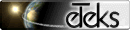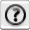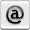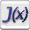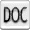# Jeks forum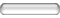This forum is dedicated to Jeks.
You may read freely the messages it contents. If you want to write a message or answer to a subject, subscribe to it first.

 Messages of subject Using comparison ameet Location : Mozambique Member since : Jul 21, 2005 Messages : 1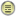Jul 21, 2005 at 6:17 PM I want to calculate the result of simple expressions like this: "45>30" "2<=3" How can I do it?---Ameet Bussard Member since : Jan 30, 2012 Messages : 1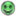Jan 30, 2012 at 4:13 PM If anybody else needs this information it can be done this way: public boolean calcBoolFormula( String formula ) throws CompilationException{ CalculatorParser parser = new CalculatorParser (new JavaSyntax()); Double result = parser.computeExpression(formula); return result.equals(DoubleInterpreter.TRUE_DOUBLE); } Usage: public static void main(String[] args) { try { System.out.println("1>2"+calcBoolFormula("1>2")); System.out.println("1=1"+calcBoolFormula("1 == 1")); System.out.println("1<2"+calcBoolFormula("1<2")); } catch (CompilationException e) { e.printStackTrace(); } }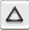© Copyrights 1997-2023 eTeks - All rights reserved